# A Monadic Model for Set Theory, Part 2

$$\newcommand{\fcd}{\leadsto}$$

Last time, we discussed funcads, which are a generalization of functions that can have multiple or no values for each element in their input.

The composition of funcads is defined to be compatible with the composition of functions, and it takes a fairly similar form:

$f :: Y \fcd Z \wedge f :: X \fcd Y \implies \exists (f \odot g :: X \fcd Z), (f \odot g)(x) = \bigcup_{\forall y \in g(x)} f(y)$

In other words, map the second function over the result of the first and take the union. If you’re a Haskell programmer, you probably were expecting this from the title and the name “funcad”: the funcad composition rule is just Haskell’s >=>.

Anyway, here are a few examples of funcad compositions (originals in blue, red. output in green.):

These compose like regular functions## Composing a funcad with its inverse

We can represent the operator:

$\star :: (A \fcd B) \to (A \fcd A)$ $\star f = f^{-1} \odot f$

### Examples

$f(x) = {x^2}$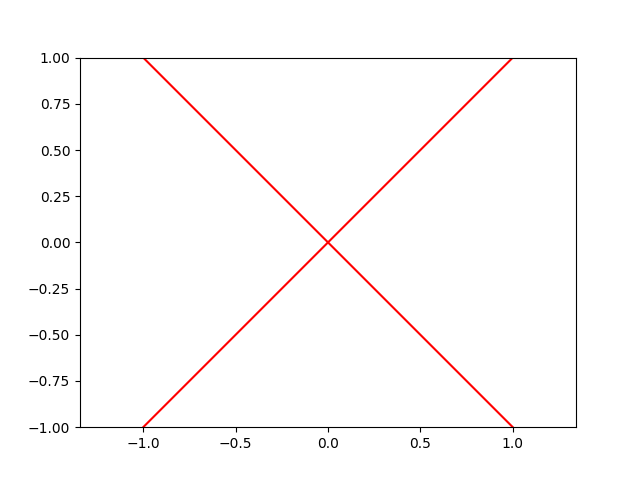$f(x) = \sqrt x$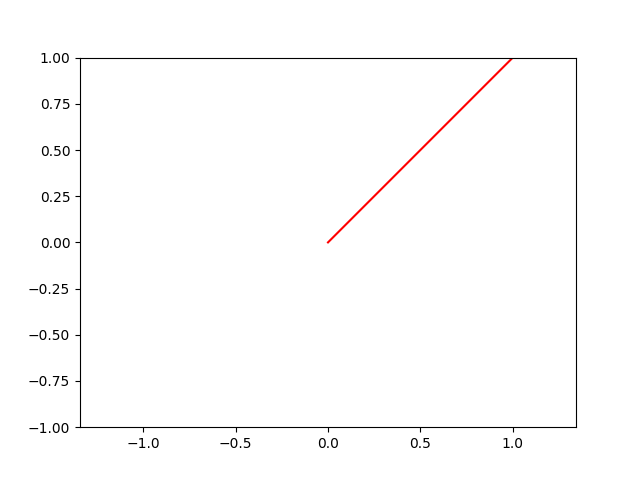$f(x) = \{y | x-1 \leq y \leq x + 1 \}$ $f^{-1}(x) = \{y | x \in f(y)\} = \{y | y - 1 \leq x \leq y + 1 \}$ $\star f (x) = \{y | y - 1 \leq z \leq y + 1, z \in \{t | x - 1 \leq t \leq x + 1\}\}$ $\star f (x) = \{y | y - 1 \leq z \wedge z \leq y + 1, x - 1 \leq z \leq x + 1\}$ $\star f (x) = \{y | y \leq z + 1 \wedge z - 1 \leq y, x - 1 \leq z \leq x + 1\}$ $\star f (x) = \{y | y \leq x + 2 \wedge x - 2 \leq y\}$ $\star f (x) = \{y | x - 2 \leq y \leq x + 2\}$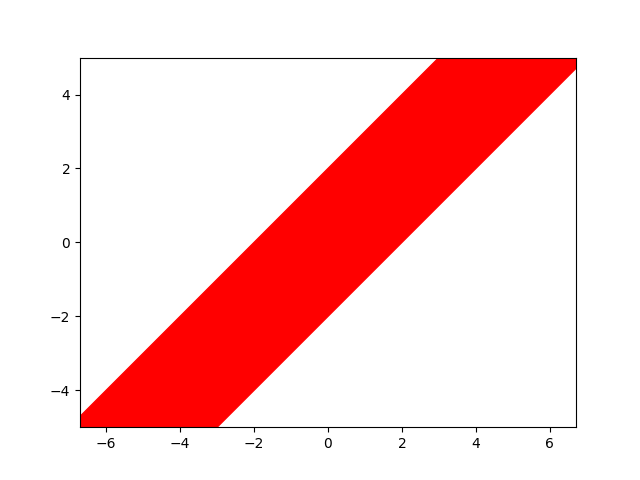### General Characterization

In general, $$\star$$ represents the well-behavedness of the given funcad under under iversion.

We can find that

$\star f(x) = f^{-1} \odot f (x) = \bigcup_{\forall y \in f(x)} f^{-1}(y)$ $\star f (x) = \bigcup_{\forall y \in f(x)} f^{-1}(y)$

Basically, $$\star f$$ represents how one-to-one $$f$$ is.

For any isomorphism $$f$$, we have $$\star f(x) = x$$.

For any one-to-one and functional funcad, we have

$\star f(x) = \bigcup_{\forall y \in f(x)} f^{-1}(y) = \bigcup_{\forall y \in f(x), |f(x)| = 1, |f^{-1}(y)| = 1} f^{-1}(y)=\left\{\begin{array}{cc} \{x\} & \text{if $$|f(x)| = 1$$}\\ \{\} & \text{otherwise} \end{array}\right.$

For example, for $$f(t) = \{t^2 - 2t\}, 0 \leq t \leq 1$$, $$\star f(t) = t, 0 \leq t \leq 1$$: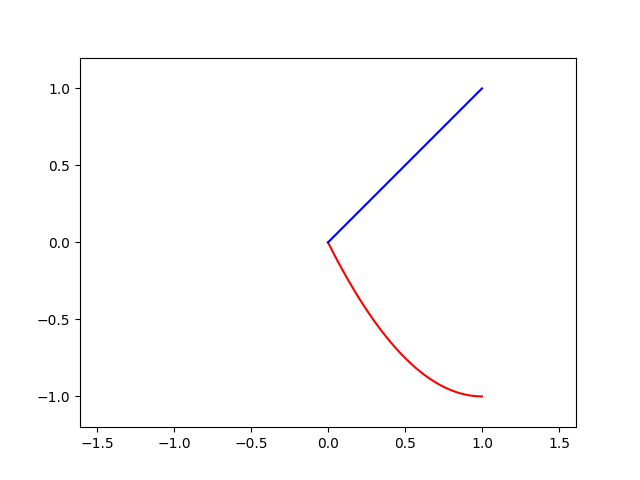For a more complex funcad, such as $$f(x) = \pm \sqrt{1-x^2}$$, we have $\star f(x) = f^{-1}(\sqrt{1 - x^2}) \cup f^{-1}(-\sqrt{1-x^2}) = \pm x, -1 \leq x \leq 1$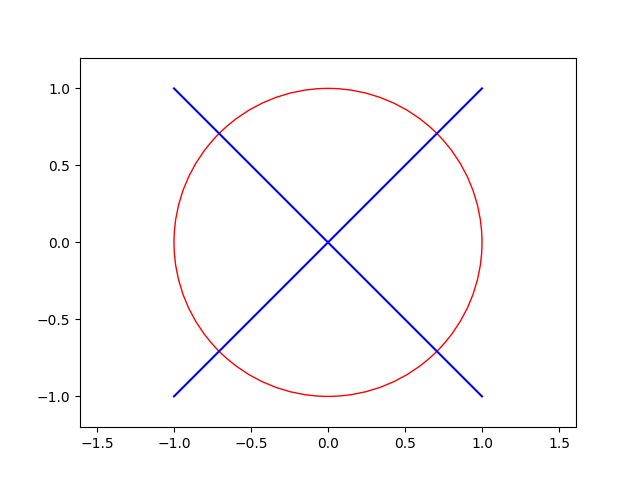## A Quick Coding Sample

So, we now know a few things about funcads. How about we put this knowledge into effect?

import Prelude hiding ((.))